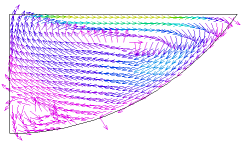﻿ Sample Problems > Applications > Fluids > buoyant

# buoyant

Navigation:  Sample Problems > Applications > Fluids >

# buoyant{  BUOYANT.PDE

This example addresses the problem of thermally driven buoyant flow

of a viscous liquid in a vessel in two dimensions.

In the Boussinesq approximation, we assume that the fluid is incompressible,

except for thermal expansion effects which generate a buoyant force.

The incompressible form of the Navier-Stokes equations for the flow of a fluid

can be written

div(U) = 0

where U represents the velocity vector,

p is the pressure,

nu is the kinematic viscosity

and   F is the vector of body forces.

The first equation expresses the conservation of momentum, while the second,

or Continuity Equation, expresses the conservation of mass.

If the flow is steady, we may drop the time derivative.

If we take the curl of the (steady-state) momentum equation, we get

Using div(U)=0 and div(curl(U))=0, and defining the vorticity W = curl(U),

we get

W.grad(U) represents the effect of vortex stretching, and is zero in

two-dimensional systems. Furthermore, in two dimensions the velocity has

only two components, say u and v, and the vorticity has only one,

which we shall write as w.

Consider now the continuity equation.  If we define a scalar function psi

such that

u = dy(psi)    v = -dx(psi)

then div(U) = dx(dy(psi))-dy(dx(psi)) = 0, and the continuity equation is

satisfied exactly.  We may write

Using psi and w, we may write the final version of the Navier-Stokes

equations as

dy(psi)*dx(w) -dx(psi)*dy(w) = nu*div(grad(w)) + curl(F)

If F is a gravitational force, then

F = (0,-g*rho) and

curl(F) = -g*dx(rho)

where rho is the fluid density and g is the acceleration of gravity.

The temperature of the system may be found from the heat equation

Dropping the time derivative, approximating rho by rho0,

and expanding U in terms of psi, we get

div(k*grad(T)) + S = rho0*cp*[dy(psi)*dx(temp) - dx(psi)*dy(temp)]

If we assume linear expansion of the fluid with temperature, then

rho = rho0*(1+alpha*(T-T0)) and

curl(F) = -g*rho0*alpha*dx(T)

--------------------------

In this problem, we define a trough filled with liquid, heated along a center

strip by  an applied heat flux, and watch the convection pattern and the heat

distribution.  We compute only half the trough, with a symmetry plane

in the center.

Along the symmetry plane, we assert w=0, since on this plane

dx(v) = 0 and u=0, so dy(u) = 0.

Applying the boundary condition psi=0 forces the stream lines to be parallel

to the boundary, enforcing no flow through the boundary.

On the surface of the bowl, we apply a penalty function to enforce a "no-slip"

boundary condition.  We do this by using a natural BC to introduce a surface

source of vorticity to counteract the tangential velocity. The penalty weight

was arrived at by trial and error.  Larger weights can force the surface

velocity closer to zero, but this has no perceptible effect on the temperature

distribution.

On the free surface, the proper boundary condition for the vorticity is

problematic. We choose to apply NATURAL(w)=0, because this implies no vorticity

transport across the free surface.    (11/16/99)

}

TITLE 'Buoyant Flow by Stream Function and Vorticity - No Slip'

 VARIABLES   temp psi w DEFINITIONS   Lx = 1   Ly = 0.5   Rad = 0.5*(Lx^2+Ly^2)/Ly   Gy = 980   { surface heat loss coefficient }   sigma_top = 0.01     { bowl heat loss coefficient }   sigma_bowl = 1           { thermal conductivity }   k = 0.0004           { thermal expansion coefficient }   alpha = 0.001     visc = 1   rho0 = 1     heatin = 10 { heat source }   t0 = 50   dens = rho0*(1 - alpha*temp)   cp = 1   penalty = 5000   u = dy(psi)   v = -dx(psi) EQUATIONStemp: div(k*grad(temp)) = rho0*cp*(u*dx(temp) + v*dy(temp))

psi:  div(grad(psi)) + w = 0

w:    u*dx(w) + v*dy(w) = visc*div(grad(w)) - Gy*dx(dens)

BOUNDARIES

region 1

{ on the arc of the bowl, set Psi=0, and apply a conductive loss to T.

Apply a penalty function to w to force the tangential velocity to zero }

start "outer" (0,0)

natural(temp) = -sigma_bowl*temp

value(psi) = 0

natural(w)= penalty*tangential(curl(psi))

{ on the top, continue the Psi=0 BC, but add the heat in put term to T,

and apply a natural=0 BC for w }

natural(w)=0

line to (0,Ly)

{ in the symmetry plane assert w=0, with a reflective BC for T }

value(w)=0

line to close

MONITORS

contour(temp) as "Temperature"

contour(psi) as "Stream Function"

contour(w)   as "Vorticity"

vector(u,v)   as "Flow Velocity" norm

PLOTS

grid(x,y)

contour(temp) as "Temperature"  painted

contour(psi) as "Stream Function"

contour(w)   as "Vorticity"  painted

vector(u,v)   as "Flow Velocity" norm

contour(dens) as "Density"  painted

contour(magnitude(u,v)) as "Speed"  painted

elevation(magnitude(u,v)) on "outer"

elevation(temp) on "outer"

END